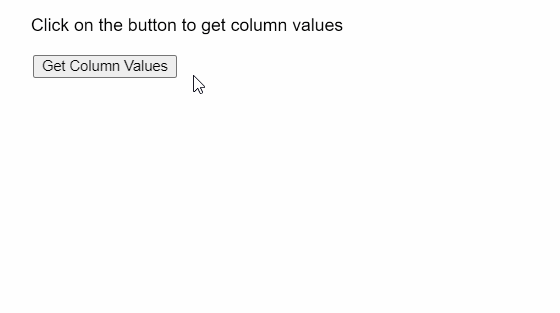Related Articles

# p5.js | p5.Table getColumn() Method

• Last Updated : 01 Jun, 2020

The getColumn() method of p5.Table in p5.js is used to retrieve all values in the given column and return them in the form of an array. The column to be returned may be given in the form of its title or ID.
Syntax:

```getColumn( column )
```

Parameters: This function accepts a single parameter as mentioned above and described below:

• column: It is a String or Number which denotes the title or the number of the column to return.

Return Value: It returns an array of column values as specified by the column parameter.
The example below illustrates the getColumn() function in p5.js:
Example:

## javascript

 `function` `setup() {``  ``createCanvas(500, 200);``  ``textSize(16);`` ` `  ``getColBtn = createButton(``"Get Column Values"``);``  ``getColBtn.position(30, 50);``  ``getColBtn.mouseClicked(getCols);`` ` `  ``text(``"Click on the button to get column values"``, 20, 20);`` ` `  ``// Create the table``  ``table = ``new` `p5.Table();`` ` `  ``// Add two columns``  ``// using addColumn``  ``table.addColumn(``"author"``);``  ``table.addColumn(``"language"``);`` ` `  ``// Add two rows``  ``let newRow = table.addRow();``  ``newRow.setString(``"author"``, ``"Dennis Ritchie"``);``  ``newRow.setString(``"language"``, ``"C"``);`` ` `  ``newRow = table.addRow();``  ``newRow.setString(``"author"``, ``"Bjarne Stroustrup"``);``  ``newRow.setString(``"language"``, ``"C++"``);``}`` ` `function` `getCols() {`` ` `  ``// Array of values in the column "author"``  ``author_col = table.getColumn(``"author"``);`` ` `  ``text(``"Column author: "``, 20, 100);``  ``// Loop through the array to display the values``  ``for` `(let i = 0; i < author_col.length; i++) {``    ``text(author_col[i], 170 + i * 120, 100);``  ``}`` ` `  ``// Array of values in the column "language"``  ``language_col = table.getColumn(``"language"``);`` ` `  ``text(``"Column language: "``, 20, 120);``  ``// Loop through the array to display the values``  ``for` `(let i = 0; i < language_col.length; i++) {``    ``text(language_col[i], 170 + i * 120, 120);``  ``}``}`

Output:Online editor: https://editor.p5js.org/
Environment Setup: https://www.geeksforgeeks.org/p5-js-soundfile-object-installation-and-methods/
Reference: https://p5js.org/reference/#/p5.Table/getColumn

Hey geek! The constant emerging technologies in the world of web development always keeps the excitement for this subject through the roof. But before you tackle the big projects, we suggest you start by learning the basics. Kickstart your web development journey by learning JS concepts with our JavaScript Course. Now at it’s lowest price ever!

My Personal Notes arrow_drop_up